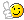# Subnetting

Junior MemberRegistered Users Posts: 2 ■□□□□□□□□□
Hi, Ill be getting similar question to this one on my exam tomorrow. Subnetting wouldn't be my strong side so If someone could go through this with a good explanation i'd really appreciate it !

Given the following network address: 186.150.0.0 Scenario: We want to have the maximum possible number of subnets but we need to accommodate at least 980 hosts per subnet. Provide the following information: The custom subnet mask.... The total and legal number of subnets.... The total and legal number of hosts per subnet....The total number of legal hosts on the entire network..... The base address for the first four legal subnets..... The broadcast address for the first four legal subnets..... The first and last host address for the first four legal subnets..... What is the subnet address for subnet 28?.... What is the broadcast address for subnet 28?.... What are the valid host addresses for subnet 28?

• Woohoo! It's over 1000! Member Posts: 1,722
So, they've given a network address without a mask. We can assume that this is a classful network. It's between 128.0.0.0 and 192.0.0.0 which makes it a class B network. This makes it a /16 network.

Know your powers of two. You can pretty much get away with knowing 2^1 to 2^8 by heart and as much more as you can. The octet powers are useful to know at least roughly, too. So, 2^8, 2^16, 2^24, 2^32

980 hosts per subnet, and make sure to consider also the network and broadcast addresses, and if specified any "room for growth". You then need to find the closest power of two that exceeds. It's 1024, which is 2^10. If you subtract 10 from 32 (the total number of bits for IPv4) you get 22, which is your CIDR subnet mask.

Now to find out how many subnet you can fit in you divide 2^22 by 2^16, which is simply two to the power of the difference between 22 and 16, which is 2^6, which is 64.

Now, as we said each subnet is 1024 addresses. This means that we increment each subnet by 1024. But each octet is 256. So, the first one is 186.150.0.0 to 186.0.3.255, the second is 186.150.4.0 to 186.0.7.255, the third is 186.150.8.0 then 186.150.12.0 and so on. What you notice is that you increment the third octet by 4 for each subnet. Another way to realise this is that you are taking 2 bits from the 3rd octet for your hosts, and 2 bits is 2^2 or 4. Or that since you have 64 subnets, you are using 6 bits for the subnets, leaving 2 bits for the hosts.

So, if each subnet increases by 4, then counting (from zero) the 28th subnet will start with 4x27 (because we start from 0), which is 108. So, the subnet address is 186.150.108.0, the broadcast address is one less than the next subnet or 186.150.111.255. This means that your range for hosts would be the remaining addresses of 186.150.108.1 to 186.150.111.254.

The quick way in your head becomes: 980 means 1024 hosts, means 2^10, means /22, means (22-16) 2^6 (64) total subnets, means a subnet every 2^2 in the third octet. Means that the nth subnet starts at 4*(n-1), meaning first 4 are 0,4,8,12 and the 28th is 108, the broadcast is one less than the next subnet (112) 111.255. The hosts are everything in between.
2017 Goals - Something Cisco, Something Linux, Agile PM
• Junior Member Registered Users Posts: 2 ■□□□□□□□□□
Thank a lot! Finally it makes some sense !
One more question " What are the valid host addresses for subnet 28" ? Would that be everything between 185.150.108.1 and 185.150.111.254 ?
Oh and what would be the total number of hosts on the entire network ? I divided 255 by 4, got 63.75 , then multiplied that by 1024 = 65280. Would that be correct ?

Thanks again !! You're a life saver !• Woohoo! It's over 1000! Member Posts: 1,722
The host addresses are everything in the subnet EXCEPT for the network address and the broadcast address. So, the total number of hosts per subnet is the whole of address space for the subnet (if it's a /22 that mean 32-22 bits for your hosts), minus those two addresses. Then multiple by the total number of subnets (the total size is 16, so 22-16 bits for your subnet addresses).

It sounds like you are doing homework, though. So, I'm not going to make it too easy. The best thing to do is just keep doing these exercises. It might take a dozen, or it might take 23 or 187, but eventually it becomes easy enough to do mostly in your head.
2017 Goals - Something Cisco, Something Linux, Agile PM